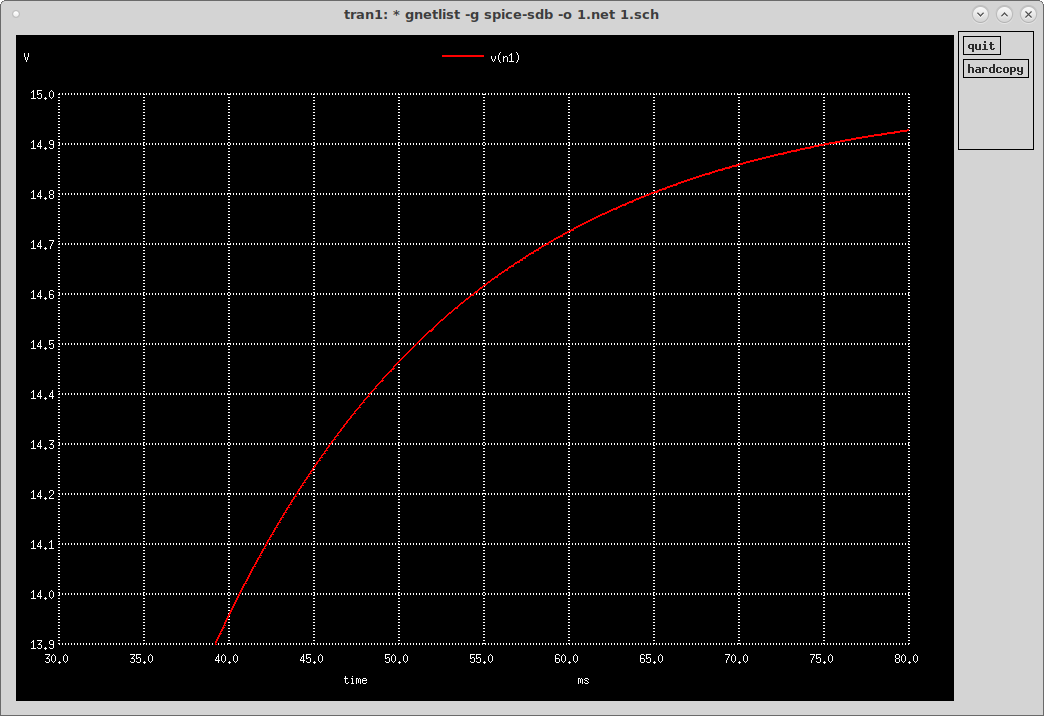# Capacitor RC Time Constant

Let's use this circuit to see the RC time constant of a capacitor. The time constant RC = 15kΩ * 1uF = 15ms.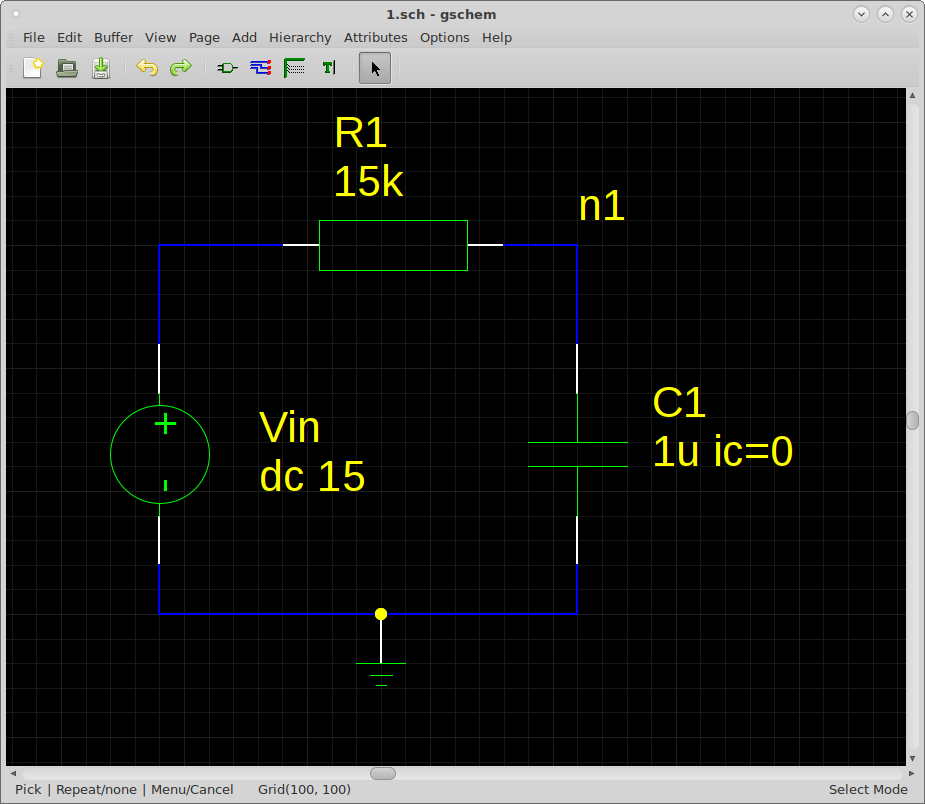Let's do a transient analysis of the circuit:

`tran 0.01m 100m uic`
(uic uses initial conditions, where the initial capacitor voltage is set to 0V by ic=0)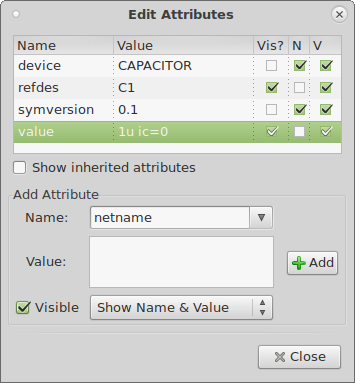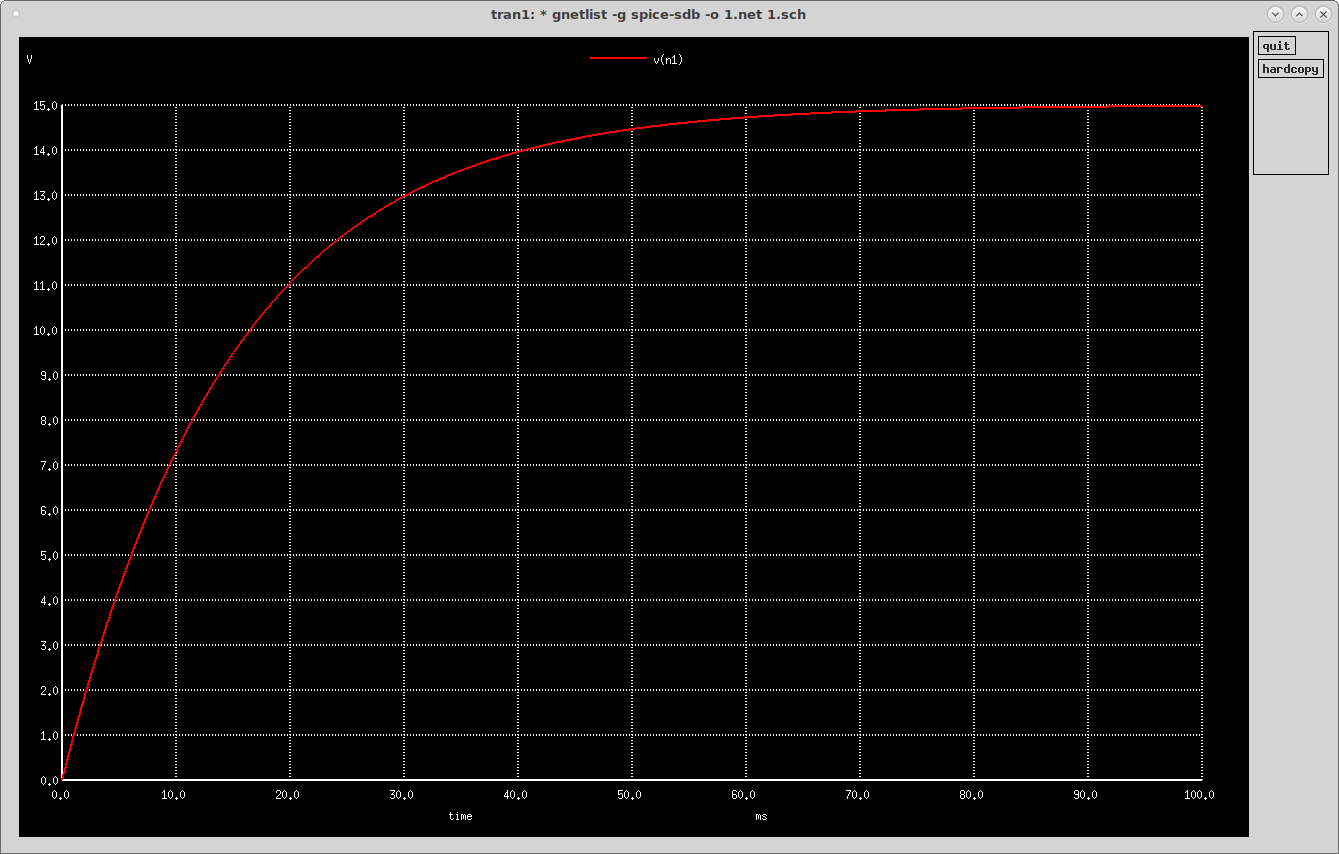We can see that in one time constant (15ms), the voltage rises to about 63% of its value! 15V * 0.63212 = 9.4818V: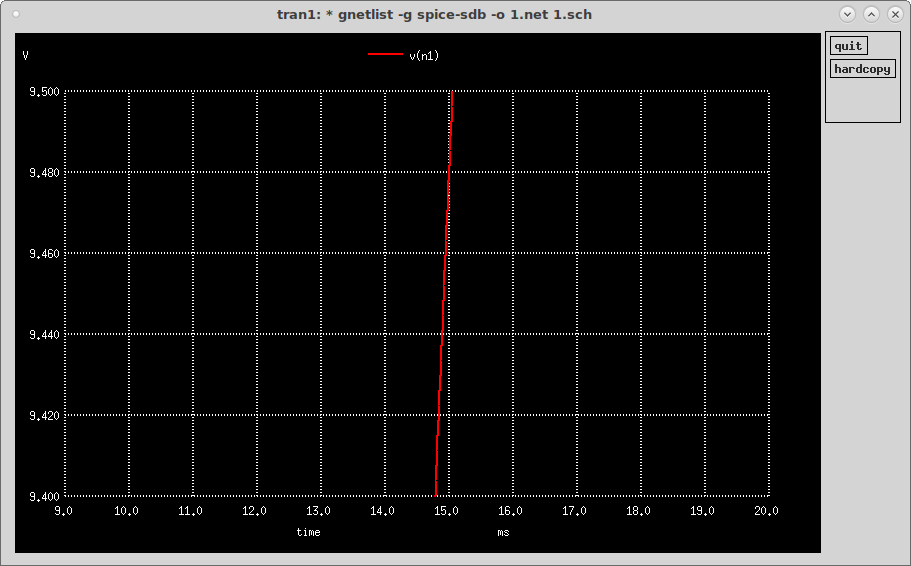We can also see that the capacitor charges to within 1% of its final value (>14.85V) in 5 time constants (75ms), the "5RC rule of thumb" (from The Art Of Electronics, Paul Horowitz, Winfield Hill, ISBN: 0-521-37095-7):